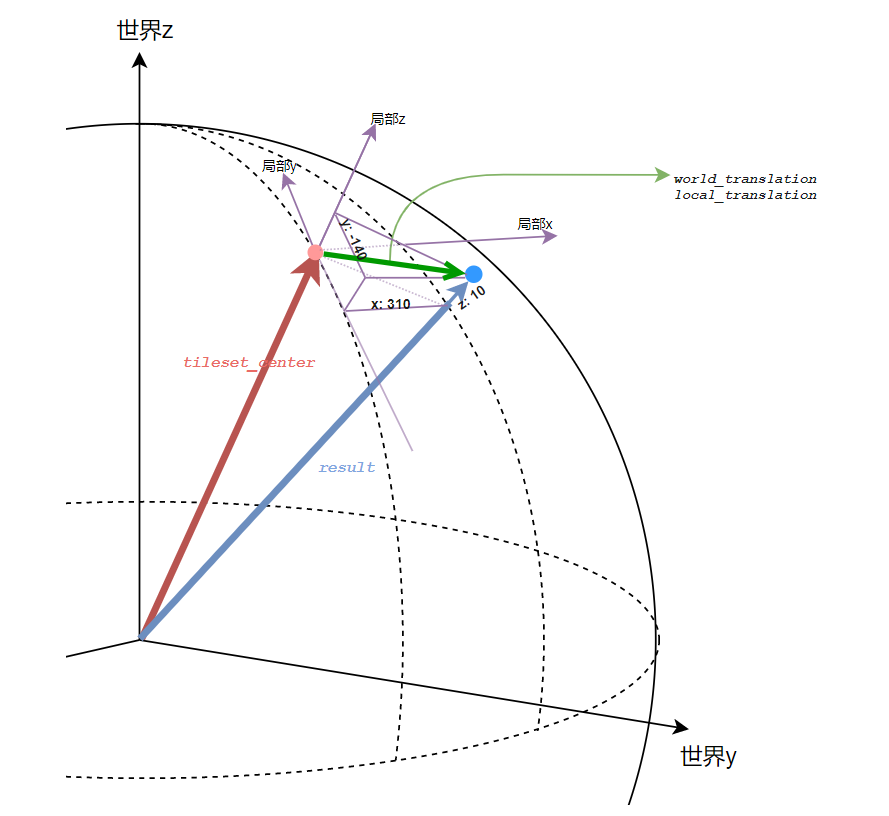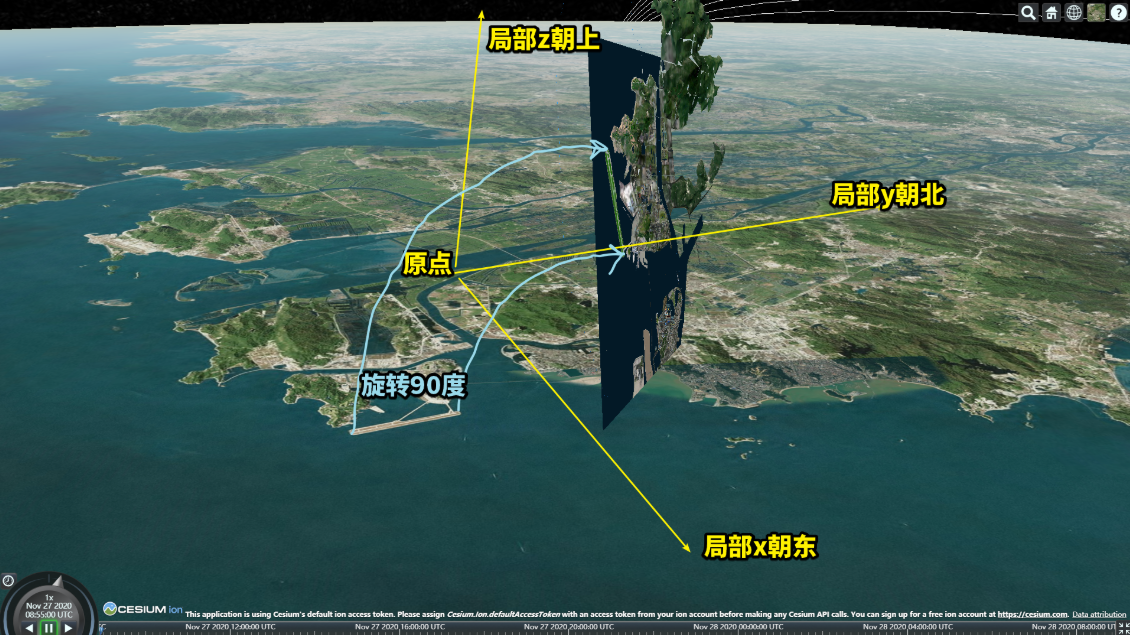# 平移思路

• 获取当前瓦片数据集的包裹范围（boundingSphere）中心（此时参考系是世界坐标）
• 计算当参考系是局部坐标时，此位置为原点的局部坐标系，到世界坐标的转换矩阵（eastNorthUpToFixedFrame）
• 利用上一步的转换矩阵，左乘一个局部平移向量，得到此平移向量在世界坐标系下的平移目标位置（矩阵×向量，结果是向量）
• 向量相减：世界坐标系下，指向平移目标点位的目标向量 - 指向数据集中心的向量，得到世界坐标系下的平移向量。
• 将世界坐标系下的平移向量转换成平移矩阵，赋予 tileset.modelMatrix

## 代码

tileset
.then(tileset => {
const tileset_center = tileset.boundingSphere.center; // Cartesian3
const frompoint_to_world_matrix = Cesium.Transforms.eastNorthUpToFixedFrame(tileset_center); // Matrix4
const local_translation = new Cesium.Cartesian3(310, -140, 10); // 向模型中心为原点，正北为y，正东为x，地心朝上为z分别平移 310、-140、10米
const result = new Cesium.Cartesian3(0,0,0);
Cesium.Matrix4.multiplyByPoint(frompoint_to_world_matrix, local_translation, result); // 转换矩阵左乘局部平移向量，结果存储在 result 中，结果是世界坐标下的平移终点向量
const targetpoint_to_world_matrix = Cesium.Transforms.eastNorthUpToFixedFrame(result);

const world_translation = new Cesium.Cartesian3(
targetpoint_to_world_matrix - frompoint_to_world_matrix,
targetpoint_to_world_matrix - frompoint_to_world_matrix,
targetpoint_to_world_matrix - frompoint_to_world_matrix,
); // 向量相减，得到世界坐标下的平移向量

tileset.modelMatrix = Cesium.Matrix4.fromTranslation(world_translation); // 构造平移矩阵并赋值
viewer.zoomTo(tileset);
});


## 图解• 红点：frompoint（地表点）
• 蓝点：targetpoint（frompoint局部坐标向东向北向上偏移各 310、-140、10米 后得到的目标点）
• 红向量：地表点向量
• 蓝向量：目标点向量
• 绿向量：平移向量。如果是局部坐标，那么就是 (310, -140, 10)，如果是世界坐标下的，那就是 蓝向量 - 红向量

cesium 的场景数据最终都是世界坐标的，所以要求的是绿向量的世界坐标表达，然后构造平移矩阵。

# 平移思路二

tileset.modelMatrix $= M_{backToOrigin}·M_{localTranslation}·M_{moveToWorldCenter}$

# 旋转思路

tileset.modelMatrix $= M_{backToOrigin}·M_{localRotate}·M_{moveToWorldCenter}$

$M_{backToOrigin}$ 则是从世界坐标中心再移动到模型原点

## 效果图（绕模型本身x轴转90度）## 代码

tileset
.then(tileset => {
const tileset_center = tileset.boundingSphere.center; // Cartesian3
//console.log(tileset_center);
const backto_matrix = Cesium.Matrix4.fromTranslation(tileset_center);
const moveto_vec = Cesium.Cartesian3.multiplyByScalar(tileset_center, -1, new Cesium.Cartesian3());
//console.log(moveto_vec);
const moveto_matrix = Cesium.Matrix4.fromTranslation(moveto_vec);

/* 绕x（即东方轴）转90度 */
const cos_rotateX = Math.cos(Math.PI/2);
const sin_rotateX = Math.sin(Math.PI/2);
const arr = [1,0,0,0,  0, cos_rotateX, sin_rotateX,0,   0,-sin_rotateX,cos_rotateX,0, 0,0,0,1];
const rotateX_matrix = Cesium.Matrix4.fromArray(arr);

/* 计算最终矩阵 */
const temp = Cesium.Matrix4.multiply(rotateX_matrix, moveto_matrix, new Cesium.Matrix4());
const r = Cesium.Matrix4.multiply(backto_matrix, temp, new Cesium.Matrix4());

tileset.modelMatrix = r; // 构造平移矩阵并赋值
viewer.zoomTo(tileset);
});


# 缩放思路

tileset.modelMatrix $= M_{backToOrigin}·M_{localScale}·M_{moveToWorldCenter}$

# 注

posted @ 2020-11-30 01:55  四季留歌  阅读(3135)  评论(4编辑  收藏  举报